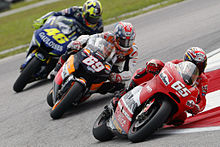# Q & A: Why does a cyclist lean inward when moving in a circular path?

Q:
Why does a cyclist bend inward when moving in a circular path? What will be the angle with the vertical considering friction? Derive the fomula?
- apurva (age 17)
A:Some serious bike leaning!

The torque about the bicycle wheels and the ground has two components: the centrifugal force  component: τ c = Fc h = mv2h/r where m is the mass of the rider plus bicycle, v is the velocity, h = the height of the center of mass of the system above the ground, and r is the radius of the circle followed by the center of mass.  The other torque is the component of gravity in the tangential direction:
τ c = mgh*tan(theta) where g is the acceleration of gravity, m and h are the same as above and theta is the angle of the tilt.  Equating the two torques and solving for theta gives:  tan(theta) = v2/rg .

If the acceleration v2/r is too big, then the maximum friction forces are not enough to counteract the centrifugal forces.  What happens then is that the bicycle will skid out from under you and you crash.

LeeH

For those who dislike using accelerated reference frames and centrifugal forces, in a standard frame that same force is the inward friction force on the tire needed to account for the inward acceleration. A vertical force of mg must act upward on the tire. Then you can just calculate torques about the center of mass.   mike w

(published on 11/10/2009)